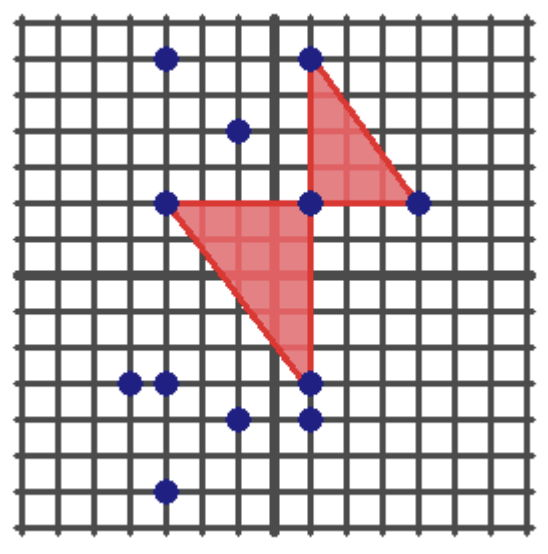시간 제한메모리 제한제출정답맞힌 사람정답 비율
2 초 512 MB222100.000%

## 문제

There are N (1 ≤ N ≤ 100 000) distinct points on a 2D plane, with the ith one at integral coordinates (xi, yi) (−109 ≤ xi ≤ 109, −109 ≤ yi ≤ 109).

A Fantastic Right Triangle is one which uses 3 of the given points as its vertices, is non-degenerate (i.e., has positive area), and which has both of its legs axis-aligned (one of its two shortest sides is horizontal and the other shortest side is vertical). The vertex at which the two legs of such a triangle meet (which has an interior right angle) is known as the triangle’s Spectacular Vertex.

A Stupendous Bowtie consists of an unordered pair of Fantastic Right Triangles which share the same point as their Spectacular Vertex, and which touch only at that point. Count the number of Stupendous Bowties which exist amongst the given points.

## 입력

The first line of the input contains one integer, N. The remaining N lines each contain two integers, xi and yi for i = 1..N.

## 출력

Output a single integer, the number of Stupendous Bowties which exist amongst the given points.

## 제한

• N ≤ 100 000
• −105 ≤ xi ≤ 105
• −105 ≤ yi ≤ 105

## 예제 입력 1

12
-3 6
1 6
-1 4
-3 2
1 2
4 2
-4 -3
-3 -3
1 -3
-1 -4
1 -4
-3 -6


## 예제 출력 1

8


The diagram below illustrates the set of given points (represented by the 12 blue dots), with one of the eight possible Stupendous Bowties shown in red.